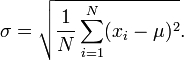# Z-score test

The fish weights in a certain lake are normally distributed with a mean of 11 lb and a standard deviation of 6. If four fish are randomly selected, what is the probability that the mean weight will be between 8.6 and 14.6 lb? Your answer should be a decimal rounded to the fourth decimal place.

p =  0.673

### Step-by-step explanation:Did you find an error or inaccuracy? Feel free to write us. Thank you!

Showing 1 comment:
Dr Math

Tips for related online calculators
Looking for help with calculating arithmetic mean?
Looking for a statistical calculator?
Looking for a standard deviation calculator?
Need help calculating sum, simplifying, or multiplying fractions? Try our fraction calculator.

#### Grade of the word problem:

We encourage you to watch this tutorial video on this math problem: